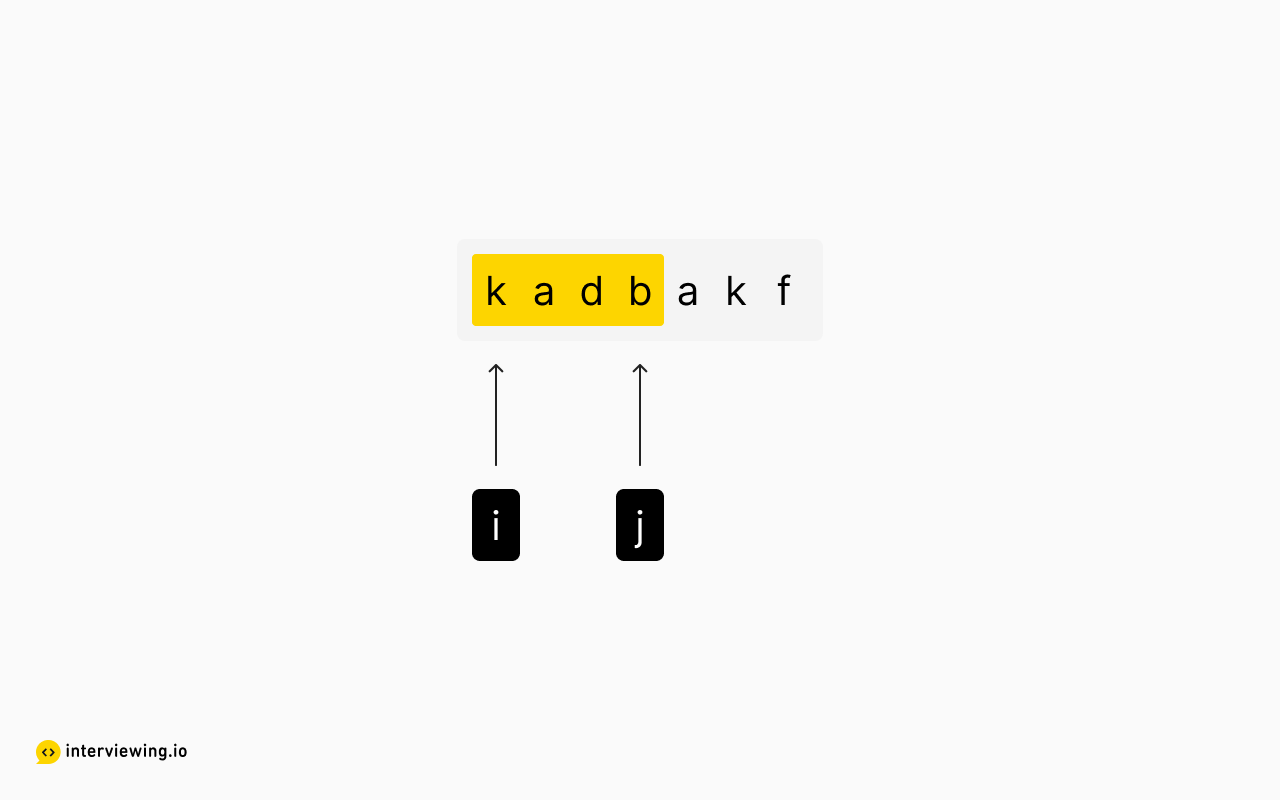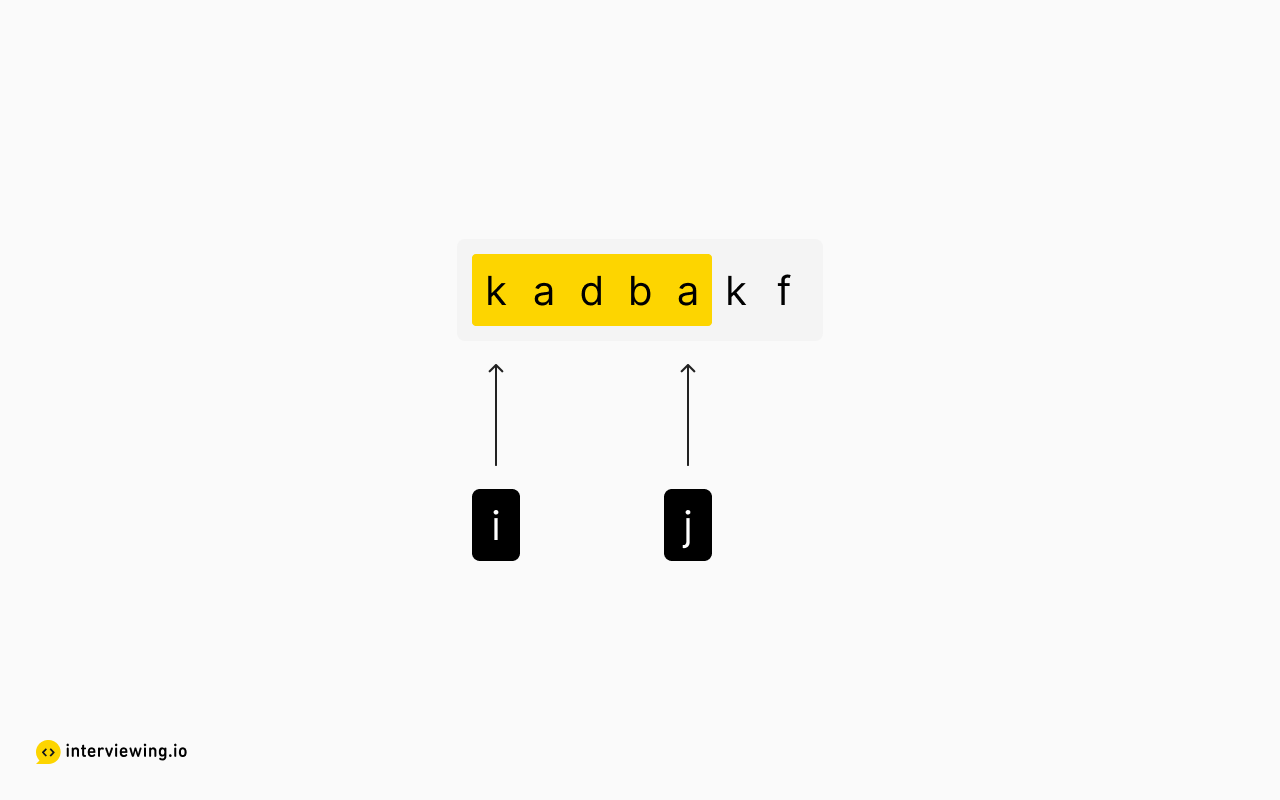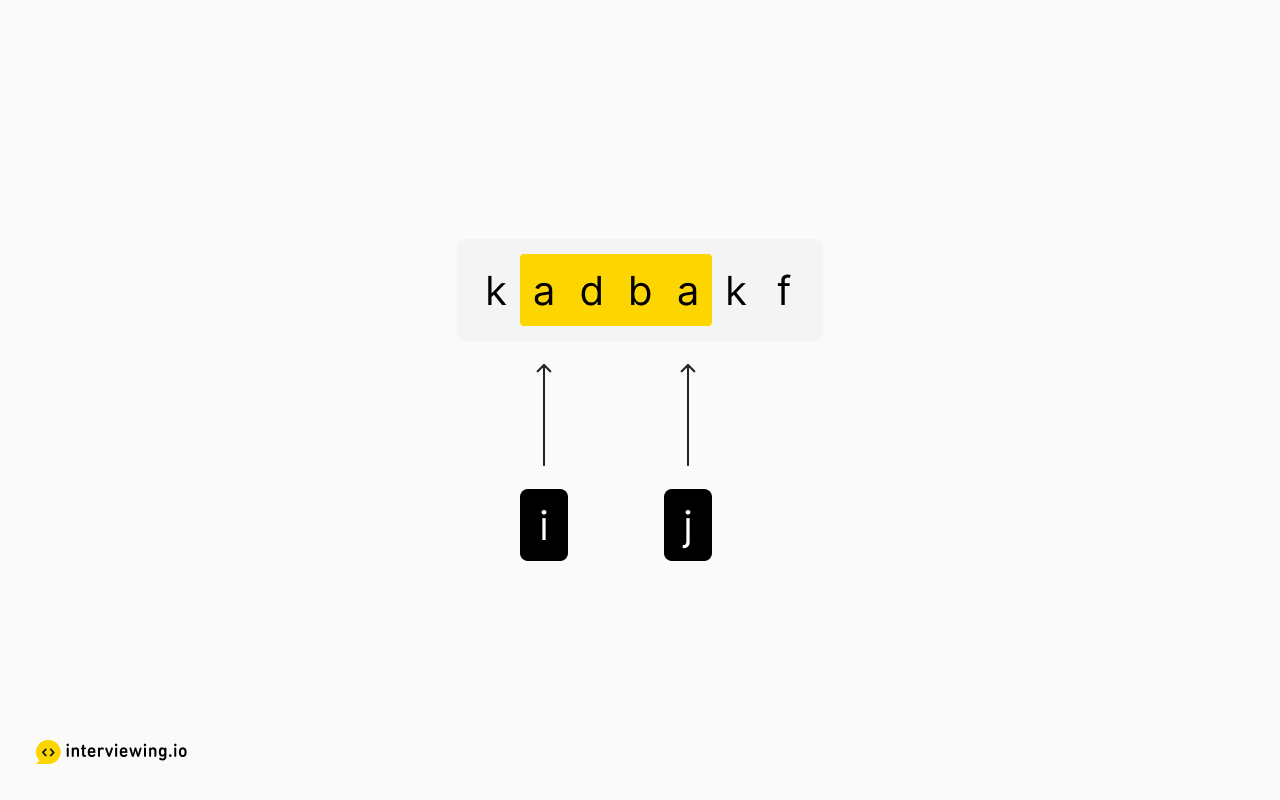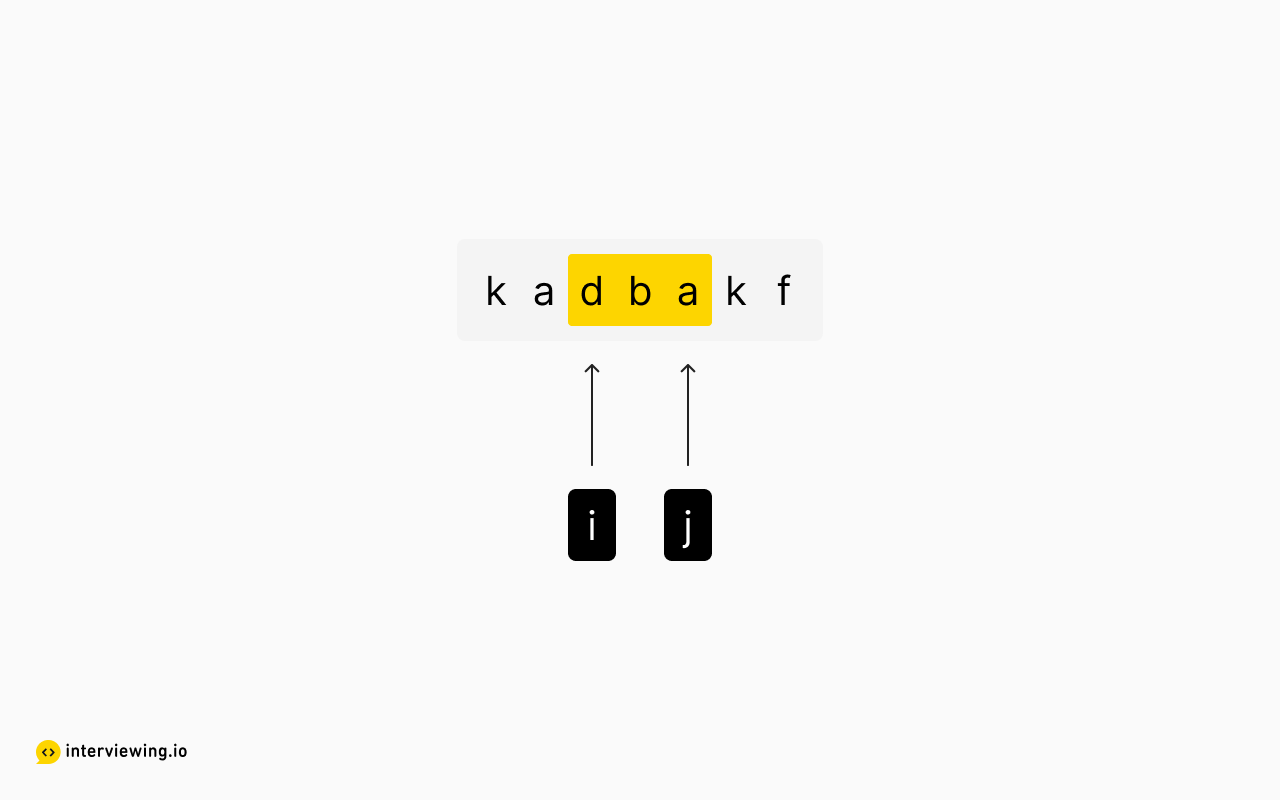MEDIUM
DATA STRUCTURES AND ALGORITHMS

# How to Solve Longest Substring Without Repeating Characters

Written ByTom WagnerKenny Polyak

## What is the Longest Substring Without Repeating Characters Problem?

The Longest Substring Without Repeating Characters problem involves searching through a given string and identifying the longest sequence of non-repeating characters. This problem can be solved with a brute force search approach, or with a more advanced and efficient sliding window technique.

## Longest Non-repeating Substring Example Inputs and Outputs

Given a string `s`, find the length of the longest substring without repeating characters.

### Example 1

Input: "abcabcbb" Output: 3 The longest substrings without repeating characters are `abc`, `bca`, and `cab`, all with length 3.

### Example 2

Input: "bbbbb" Output: 1 The longest substring without repeating characters is `b`, with length 1.

### Example 3

Input: = "pwwkew" Output: 3 The longest substring without repeating characters is `wke`, with length 3.

Constraints

• 0 <= s.length <= 1000000
• The character set for `s` is ASCII.

## Solution to the Longest Substring Without Repeating Characters Interview Question

There are three ways to approach the longest substring problem. Before we talk about them in detail, note that when asked to explore a "subsection" of an array or string, you should always confirm your understanding of the search space. Remember that a substring is a contiguous slice of characters taken from a string (similar to a subarray), as opposed to a subsequence which can skip characters, or a permutation which can be out of order. With this knowledge, we can rule out any need for backtracking, and instead start to think more greedy.

Another important thing to confirm with your interviewer when handling strings is the character set, as this will help us define the space complexity of the problem. A safe assumption is that our solution will implement ASCII (128 characters) or Extended ASCII (256 characters).

Now, here are the three approaches you can take.

### 1. Brute Force

With the search space defined, a naïve approach becomes fairly straightforward: generate each substring from the input string, check if there are any repeating characters for each, and use a standard-library function (such as `math.max()` in Java) to record the max length found for valid substrings.

We can separate this work into two subproblems. First, we need a helper function to determine if a given string has any repeating characters - this can be done by scanning a given range and counting character occurrences with a hash map.

Next, to generate each substring from the input string `s`, we can enumerate all substrings with two nested loops. Given `i` and `j` as all possible start and end indices for a substring of `s`, we have `0 <= i <= j <= n`, where `n` is the length of the input string.

### Longest Substring Without Repeating Characters Python and JavaScript Solutions - Brute Force

``````class Solution:
def is_unique_within_range(start: int, end: int) -> bool:
chars = set()
for i in range(start, end + 1):
char = s[i]
if char in chars:
return False
return True
def length_of_longest_substring(s: str) -> int:
result = 0
for i in range(len(s)):
for j in range(i, len(s)):
if (self.is_unique_within_range(i, j)):
result = max(result, j - i + 1)
return result``````

#### Time/Space Complexity Analysis

• Time Complexity: O(n³). Two nested loops is O(n²), and the helper function makes an additional nested loop.
• Space Complexity: O(min(n, m)), where `n` is the length of the string `s` and `m` is the size of the character set.

### 2. Sliding Window

To optimize the naïve approach, let's see where duplicate work is being performed.

Consider this example: if the string "abc" has no repeating characters, and we append a new character "d" at the end to make the string "abcd", do we need to re-check if "abc" has repeating characters? Our two nested loops are doing just that - repeatedly checking sections of the string that have already been evaluated - and our helper function is re-checking for repeat characters without using information from overlapping checks.

Instead of naively enumerating each substring, how can we intelligently skip substrings that we already know to be invalid? Let's use the string “kadbakf” as an example.`kadb` is a valid candidate for longest substring since it has no repeating characters.If `j` moves to index 4 though, the new substring `kadba` would be invalid. So, we can deduce that with `i` at position 0, all subsequent iterations of `j` will be invalid, since they will contain this invalid substring. We can confidently stop iterating `j` at this point.So, let's iterate `i` and begin considering new candidate substrings, since we've already seen the longest possible substring with `i` at index 0. But `adba` is also invalid, so any iteration on `j` will remain invalid.Let's increment `i` until the substring in the range `i` to `j` is valid again. Here, once `i` is at index 2, there is only one `a` character in the substring, so we can again try to increase the length by iterating `j` anew.

This technique is known as a sliding window, and it is approach applicable to a wide variety of leetcode / algorithms problems. The window represents a candidate substring bounded by a start and an end pointer, which expands and contracts based on what we're looking for. Sliding windows are commonly used when searching for an optimal range in an iterable data structure like a string or an array. This is because the algorithm will first search for a possible answer before then expanding (or contracting, whichever is the priority) to try to optimize. For example, if we were searching for the shortest substring that contains the letters `a` and `b`, we would only contract our window if those letters existed in the current substring (valid condition), otherwise we're adding characters until the condition is met.

Conversely, when looking for the maximum possible length, as in the problem at hand, we can only expand the window when the current substring is valid (otherwise, we must first arrive at a valid state by removing characters from the left). Looking back at the example above, `j` only iterates forward while the condition is satisfied - in this case, all characters between `i` and `j` are unique - and `i` only iterates forward while the condition is not satisfied.

Having made this insight on our substring enumeration, we can further improve the way we check for substrings with unique characters. Remember that a sliding window allows us to take advantage of overlapping subproblems - so, instead of re-checking each window for distinct characters, let's track the current character count of the window and evaluate the new state when a single character is added or removed.

More formally, if we know that a substring in the range `i` to `j` has no repeating characters, then when adding the next character at `j+1`, we simply need to check if that character already exists in the previous range. And because we established the character set at the beginning of our solution, this can be determined in constant time using an array of length 128 to represent character occurrence in a substring.

Note that, although there are still two nested loops in our code, the time complexity of the iteration is now linear, as `i` and `j` will only iterate over each character once. Unlike our naïve implementation, the two pointers are not dependent on one another, and instead iterate based on the state of the range between them, which can be determined in constant time and space.

#### Longest Substring Without Repeating Characters Python and JavaScript Solutions - Sliding Window

``````class Solution:
def length_of_longest_substr(s: str) -> int:
# List representing ASCII characters to track occurences
chars =  * 128
result = 0
i = 0
j = 0
while j < len(s):
right_char = s[j]
chars[right_char] += 1
while chars[right_char] > 1:
left_char = s[i]
chars[left_char] -= 1
i += 1
result = max(result, i - j + 1)
return result``````

#### Time/Space Complexity Analysis

• Time Complexity: O(n). At worst, the two pointers both perform linear scans of the string, which would be O(2n). This can be interpreted as O(n) in Big-O notation, since constants are ignored when determining order of magnitude.
• Space Complexity: O(1). Limited to 128 ASCII characters, space is constant.

### 3. Sliding Window Optimized

As a marginal optimization, we can avoid two passes with our pointers by improving how we cache character occurrences and update our pointers.

Remember what we determined in the previous approach: once a duplicate character is found in a given window, this candidate substring will remain invalid until one of the dupes is removed. Seeing that we need to arrive at a valid state before we can continue iterating our right pointer, can we improve how we iterate the left pointer? Indeed! Instead of iteratively searching for the next valid position, we can deduce where it would necessarily be: the index directly after the first occurring duplicate's index in the substring. Moving `i` to this position effectively removes the duplicate from the substring.

Tracking character counts in our hash map won't serve us anymore - instead, let's record the last index at which each character was encountered, so the left pointer can be updated in constant time. Thus when the right pointer finds a repeat, we simply update the left pointer to the position of the last occuring repeat (plus 1).

#### Longest Substring Without Repeating Characters Python and JavaScript Solutions - Sliding Window Optimized

``````class Solution:
def length_of_longest_substr(s: str) -> int:
result = 0
hash_map = {}
i = 0
j = 0
while j < len(s):
char = s[j]
# If a duplicate is found, update i to our stored next valid position
if char in hash_map:
i = max(hash_map[char], i)
result = max(result, j - i + 1)
# Store the next index for this character, as this will be the next valid position to de-duplicate
hash_map[char] = j + 1
return result``````

#### Time/Space Complexity

• Time Complexity: `O(n)`
• Space Complexity: `O(1)`

## Practice the Longest Substring Without Repeating Characters Problem With Our AI Interviewer

Start AI Interview

## Longest Substring Without Repeating Characters Frequently Asked Questions (FAQ)

### How do you find the longest substring without repeating characters?

There are three ways to solve this problem. First, there’s the brute force approach, where you generate each substring from the input string, check if there are any repeating characters for each, and record the max length found for valid substrings. This approach runs in `O(n³)` time. A more efficient way to do it is with a sliding window approach, where you look at substrings bounded by a start and an end pointer, which expand and contract. To start, you create a new set where you track what characters you’ve seen. If the next character is not already in the set, you add it to the set and expand your window by moving the end pointer to the right. If the next character is already in the set, you remove the character at the beginning of the window from the set and contract the window by moving the start pointer to the right. At each step, you calculate the length of the current window and compare it to the previous maximum length. This approach runs in `O(n)` time. Finally, there’s the optimized sliding window approach, where you can avoid two passes by improving on how you cache character occurrences and update your pointers. This approach also runs in linear time.

### What’s the most efficient way to find the longest substring without repeating characters?

The most efficient solution to this problem is an optimized sliding window approach.